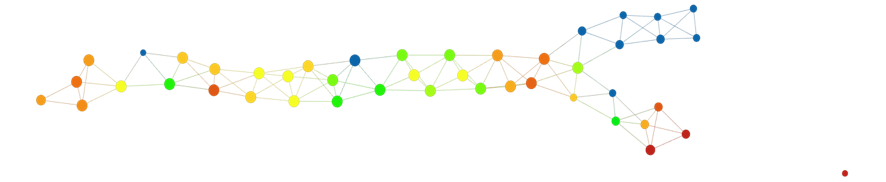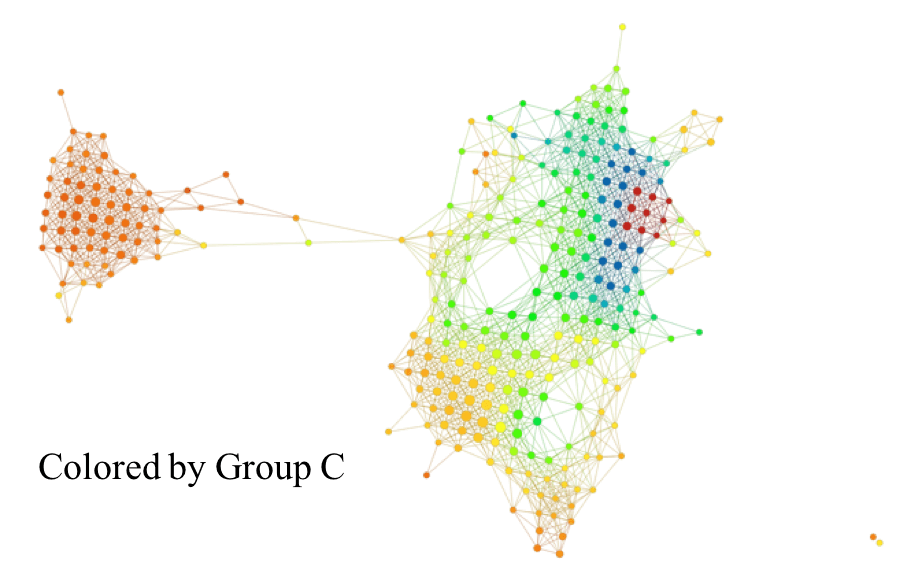# Machine Intelligence for Statistical Inference and Human Interpretation of Data

One of the criticisms of machine learning and artificial intelligence approaches to the study of data is that both are “black box” technologies, which can provide useful automated answers but which do not provide human interpretable output, and for which it is often not possible to understand how they are doing what they are doing.

Ayasdi’s approach to this problem draws upon our core technology, Topological Data Analysis (TDA) and is able to supply powerful, detailed explanations outputs. In this post, however, we will extend our work beyond the current TDA “comparison” methodology. The current methodology uses the topological networks built from the data points (rows) in a data set. In this new work, Ayasdi will also incorporate the features (columns), demonstrating an improved and readily interpreted result.

Let’s first describe how the explain methodology works.

We suppose that we have a data set, and have identified some groups in it. The groups may have been an integral part of the data (imagine a situation where a disease exists in many distinct forms, such as inflammatory bowel disease, or where one has a survivor/non-survivor information), or they may have been created by segmentation or hot spot analysis from a topological model of the set of rows.

If we select two of the groups, the Ayasdi technology permits one to produce a list of the features, ordered by their Kolmogorov-Smirnov (KS) score. Each feature has two distributions – one for each of the two groups. The KS score measures the difference between those two groups. Associated with this construction are also p-values in the standard statistical sense.

The interpretation is that the first variable is the one which best distinguished between the groups, and the remaining features are ordered by their ability to distinguish. The output of the explain mechanism is therefore an ordered list of features, and it is often possible to look through the list and obtain useful interpretations of what distinguishes the groups.However, the list is often complicated to interpret. Like a long list of responses to a Google query, one may see one particular phenomenon distributed disproportionately at the top of the list, and never see the lower responses.  What can we do to further enhance the transparency and comprehensibility of these “comparison tables”?

It is important to remember that the topological models Ayasdi constructs assumes that we are given a data matrix, and a dissimilarity or distance function on the rows of the data set. Often, that distance function can be Euclidean distance, but other choices include correlation distance and various kinds of angle distances. When one has a data matrix M, one can transpose it to a new matrix MT, where the columns of the initial matrix are now the rows of the transposed matrix, and vice versa. The process is illustrated for a small matrix A below.Having performed this operation, it is possible to construct a topological model for the set of rows of MT, i.e. the columns of the original matrix M. One has various choices for distance functions on this set.  We won’t dwell on this, but suffice it to say that the generic choices available for the rows of any data matrix are also available for this new matrix.

Now suppose that we have a data matrix M, and a group G within that data set that has been selected as we discussed above, either by a priori information or by segmentation within a topological model of the rows of M. For each column ci of M (i.e. row of MT), we can now compute the average value of the entries of ci which belong to rows belonging to G.

We will write fi,G for this value, and observe that as this number ranges over i, we obtain a function on the set of rows of MT. So, to reiterate, a group of rows of M gives rise to a function on the set of rows of MT. One of the capabilities of the Ayasdi topological models is the ability to color nodes of the topological model by the average value of functions on the rows of a data matrix over the rows corresponding to the node in question. This is an extremely useful approach to understanding properties the data.  In particular, we can now use the coloring by a group G within the row set of MT to see what characterizes the group.

Let’s look at an example.

There is a data set constructed by the Netherlands Cancer Institute (NKI), consisting of microarray analysis of samples from 272 breast cancer patients. Microarray analysis in this case provides a messenger RNA expression level from each gene in a set of genes selected for the study. From among these genes, we have selected the 1500 genes whose expression levels are the highest. We obtain a 272 1500 matrix, with the 1500 columns corresponding to 1500 genes that have the most variance across the data set, and the 272 rows corresponding to the samples. For this data set, topological analysis on the set of rows of the data matrix has already been carried out in  and .

One topological model is shown below.You will notice that it consists of a long trunk, and then splits into two smaller flares. Within the data set, there is a binary variable called eventdeath which is = 0 if the patient survived the length of the study, and is = 1 if the patient did not survive.  It is interesting to see how the survival corresponds to the structure of the graph, and one way to do that is to color by the average value of the variable eventdeath. The result of doing this is shown below.We see that the upper flare is dark blue, indicating low value of eventdeath, in fact the value is zero – meaning everyone survived. The lower flare, on the other hand, has a much worse survival rate, and the nodes at the tip consist almost exclusively of patients who did not survive.  We’d like to understand this phenomenon, to see what features in the data are relevant to creating the flares, and therefore the very different behaviors of the variable eventdeath. In order to do this, we can select various groups from the topological model.Group A is the high survival group mentioned above, Group B is the group with low survival, and Group C can be characterized as the group most distinct from the two others (a determination made visually based on the distance between the groups). Given these three groups, we can create three functions, as above, on the set of 1,500 features.

If we build a topological model of the set of features, we may color it by the average value of each of these functions. The three pictures below show what happens when we carry that out.In the comparison between the Group A and B colorings, we see a strong distinction, in that there are regions in the Group A coloring that are bright red while the corresponding region in the Group B coloring is bright blue.  Below, the left hand model is the Group A coloring and the right hand model is the Group B coloring.Groups I and II are clearly colored differently in the two colorings, with I predominantly red in the Group A coloring and predominantly blue in the Group B coloring, with the exception of a small solid region. Group II has the reverse behavior, blue in Group A and red in Group B. It is likely that these groups are correlated with high estrogen receptor expression, positively correlated in the case of Group I and negatively correlated in the case of Group II. Estrogen receptor expression is well known to be the “strong signal” for survival in breast cancer. If we compare all three groupsas in the picture, we can also see that Group C appears to be a “weaker” form of Group B, with a smaller blue region in the upper right, and a weaker red in the lower region.  It also shows a somewhat stronger red coloring on the left hand “island” than either Group A or Group B.  It would be very interesting to understand which genes are involved in the strong red group in the upper right, which persists through all three, and also which genes are involved in the left hand island. Understanding these gene sets requires the use of various web based tools for biological pathway analysis.

In summary, we have shown how to use topological modeling for the space of features in a data set rather than the set of rows to give direct insight into the data set.  A data set with more than four features cannot be directly understood visually using standard graphing techniques, but data sets with hundreds or thousands of features can be readily understood in this way.  The method gives immediate recognition of groups of features acting in concert, which is what generally happens in the analysis of genomic and more generally biological data.

 M. Nicolau, A. Levine, and G. Carlsson, Topology based data analysis identifies a subgroup of breast cancers with a unique mutational profile and excellent survival, Proc. Natl. Acad. Sci., vol. 108, no. 17, 7265-7270, (2011).

 P. Lum, G. Singh, A. Lehman, T. Ishkhanov, M. Vejdemo-Johansson, M. Alagappan, and G. Carlsson, Extracting insights from the shape of complex data using topology, Scientific Reports 3, Article number 1236,  (2013).